# RS Aggarwal Solutions for Class 7 Maths Chapter 17 Constructions

RS Aggarwal Solutions for Class 7 Maths Chapter 17 – Constructions are provided here. To cover the entire syllabus in Maths, the RS Aggarwal is an essential material as it offers a wide range of questions that test the students understanding of concepts. Our expert personnel have solved the problems step by step with neat explanations. Students who aspire to score good marks in Maths, then refer RS Aggarwal Class 7 Solutions.

Expert tutors at BYJU’S have prepared the RS Aggarwal Solutions for Class 7 Maths Chapter 17, and it contains all exercises. Students gain more knowledge by referring to RS Aggarwal Solutions. Download pdf of Class 7 Chapter 17 in their respective links.

## Download PDF of RS Aggarwal Solutions for Class 7 Maths Chapter 17 – Constructions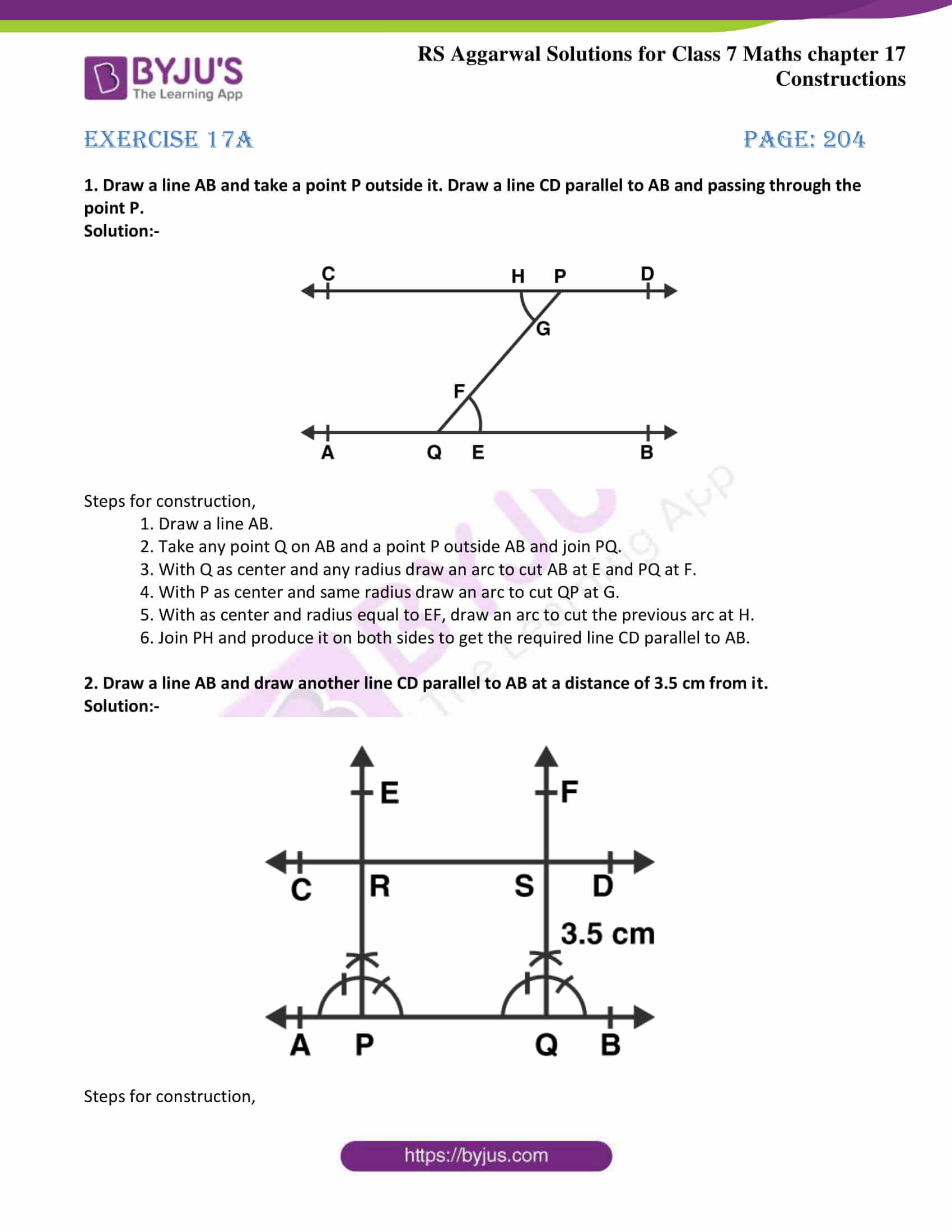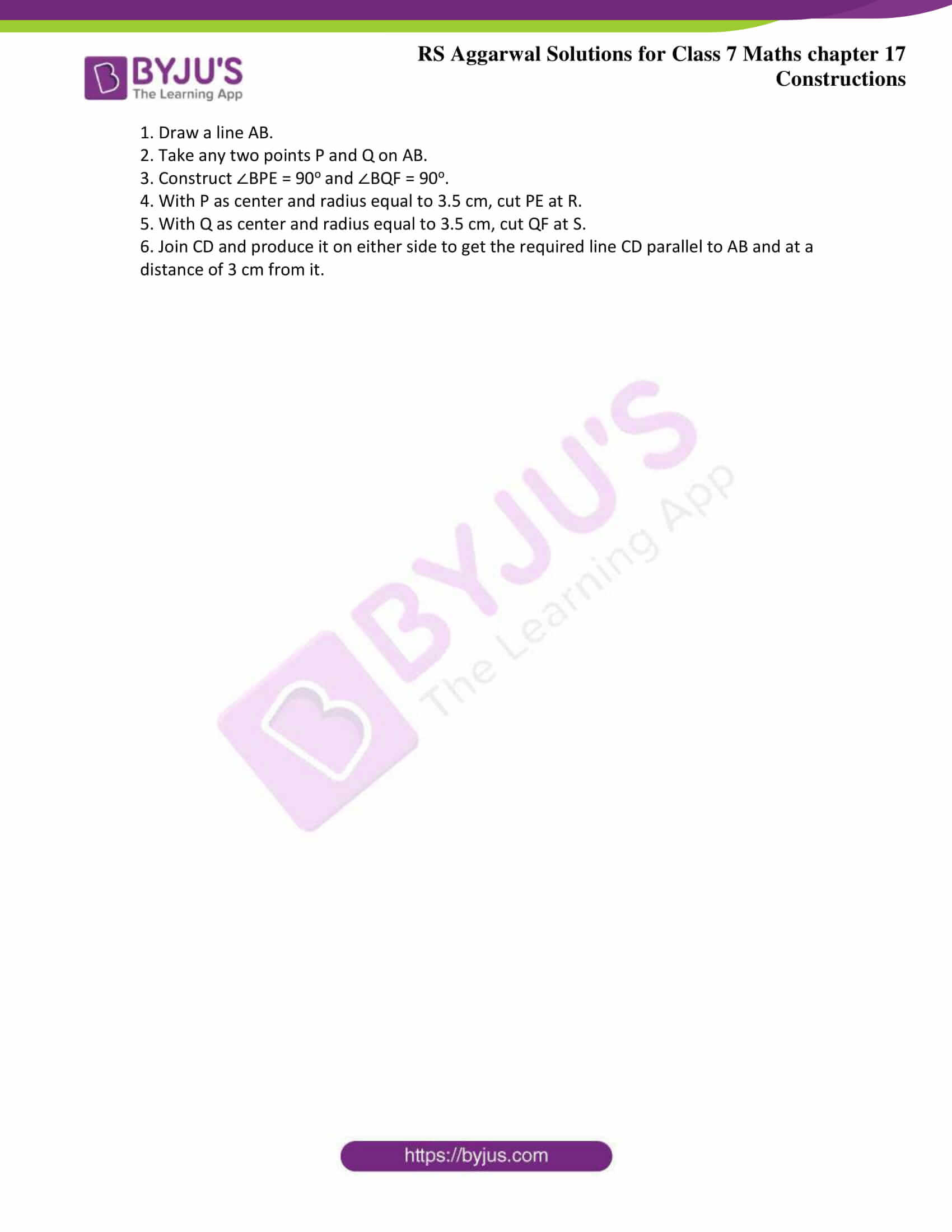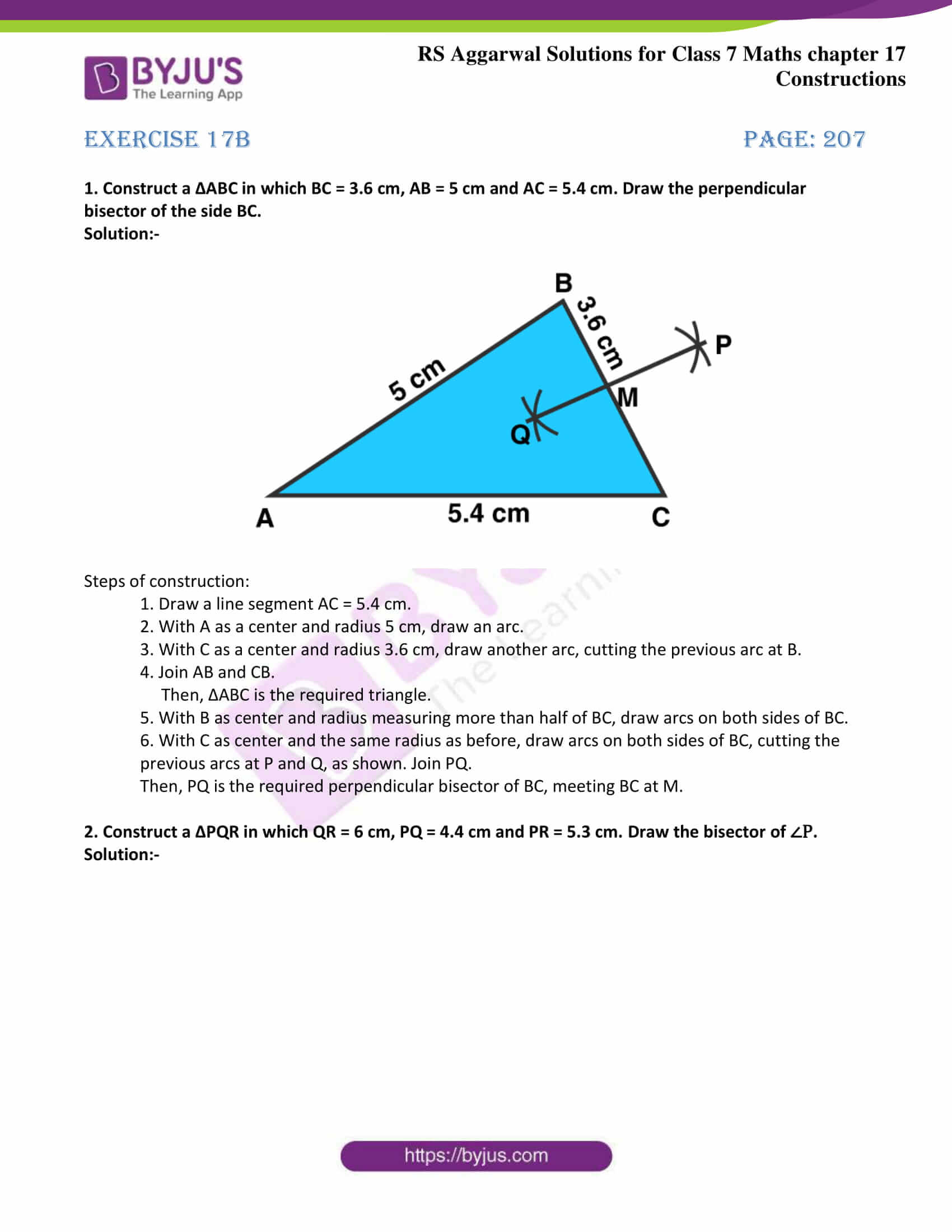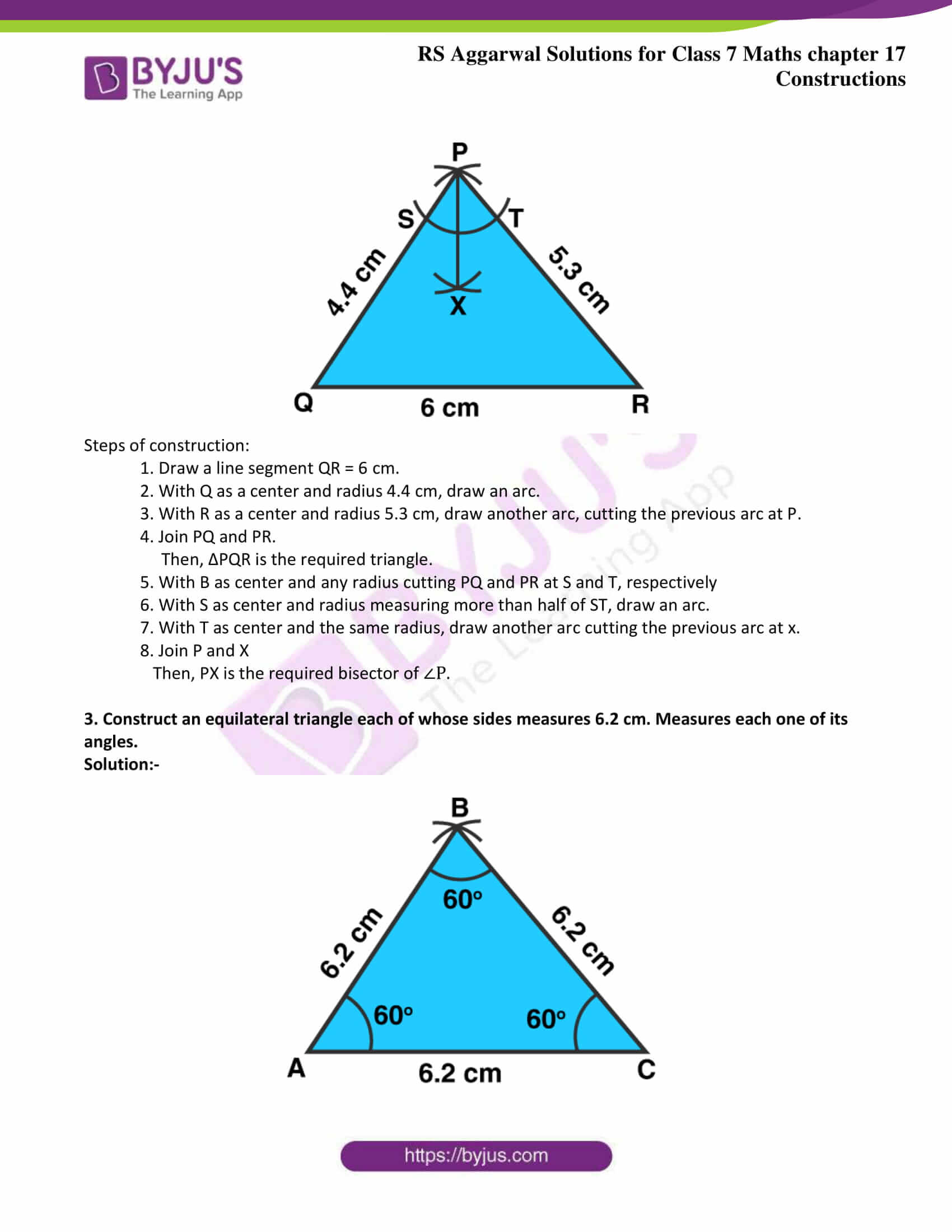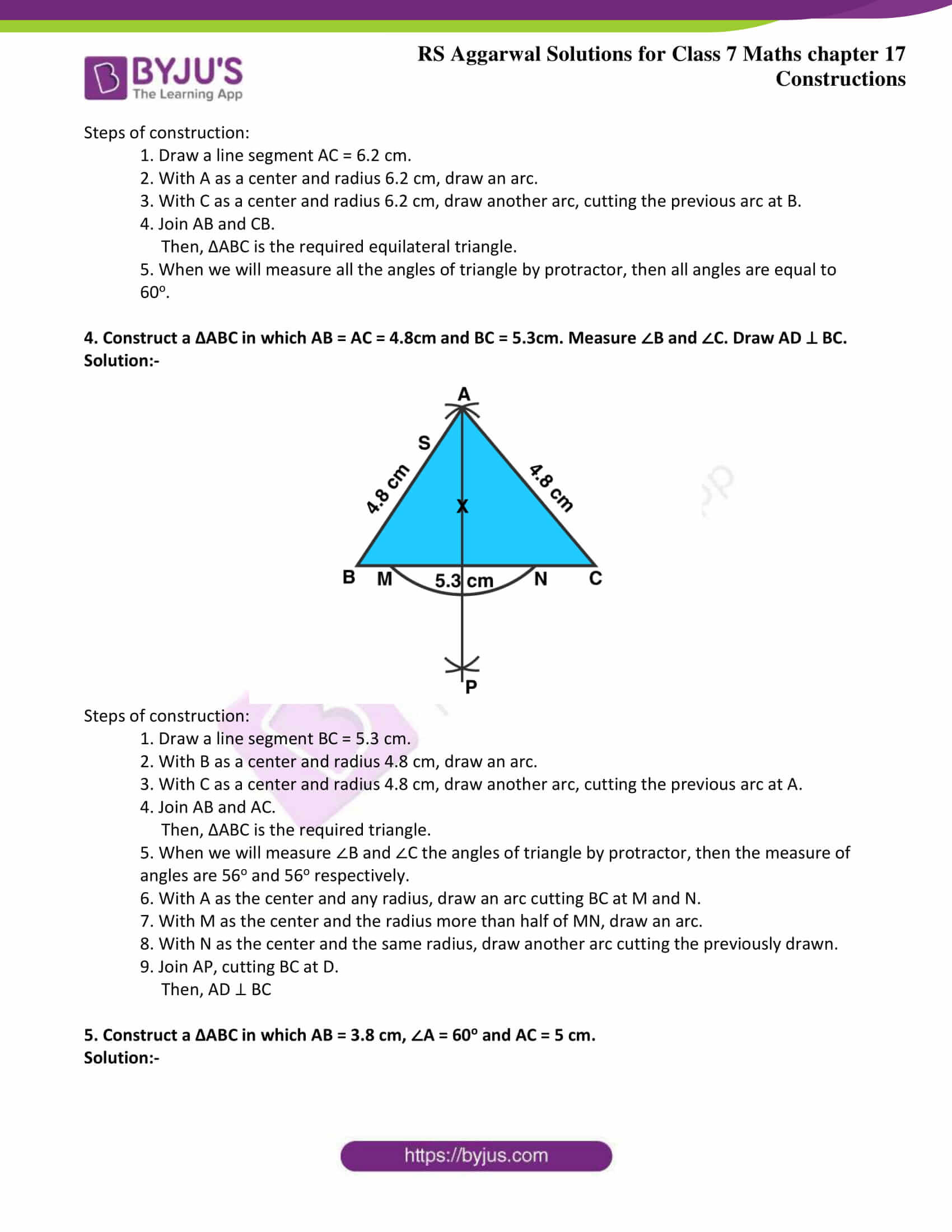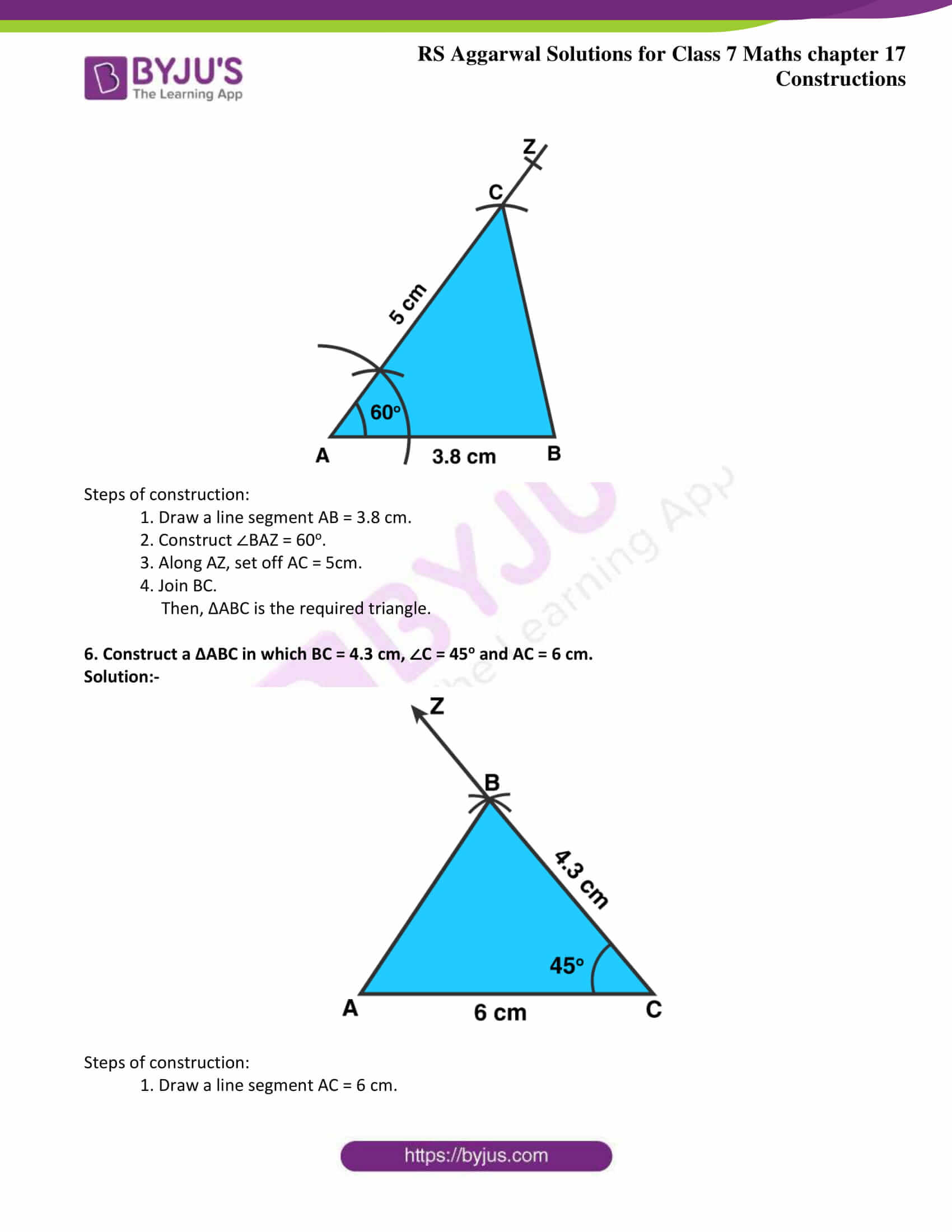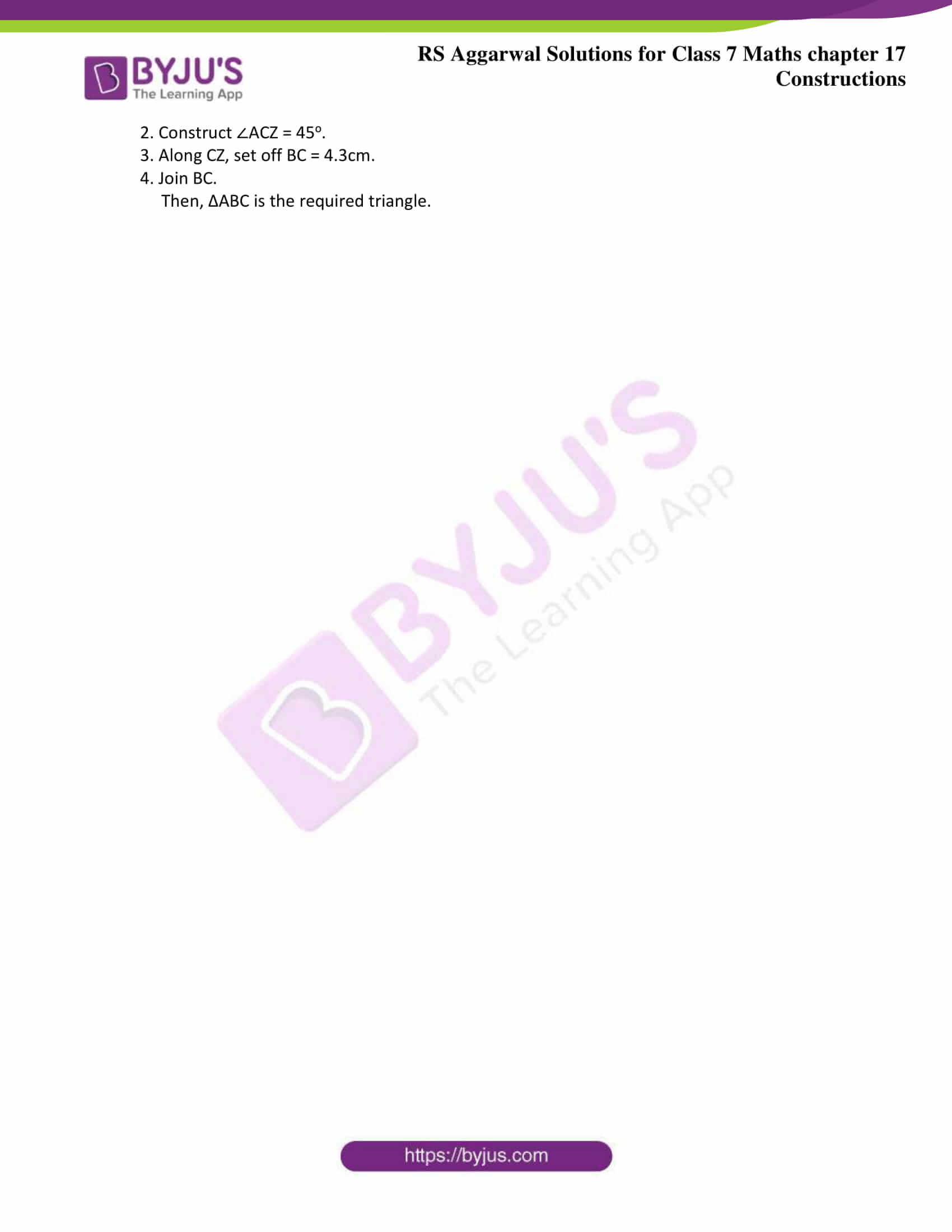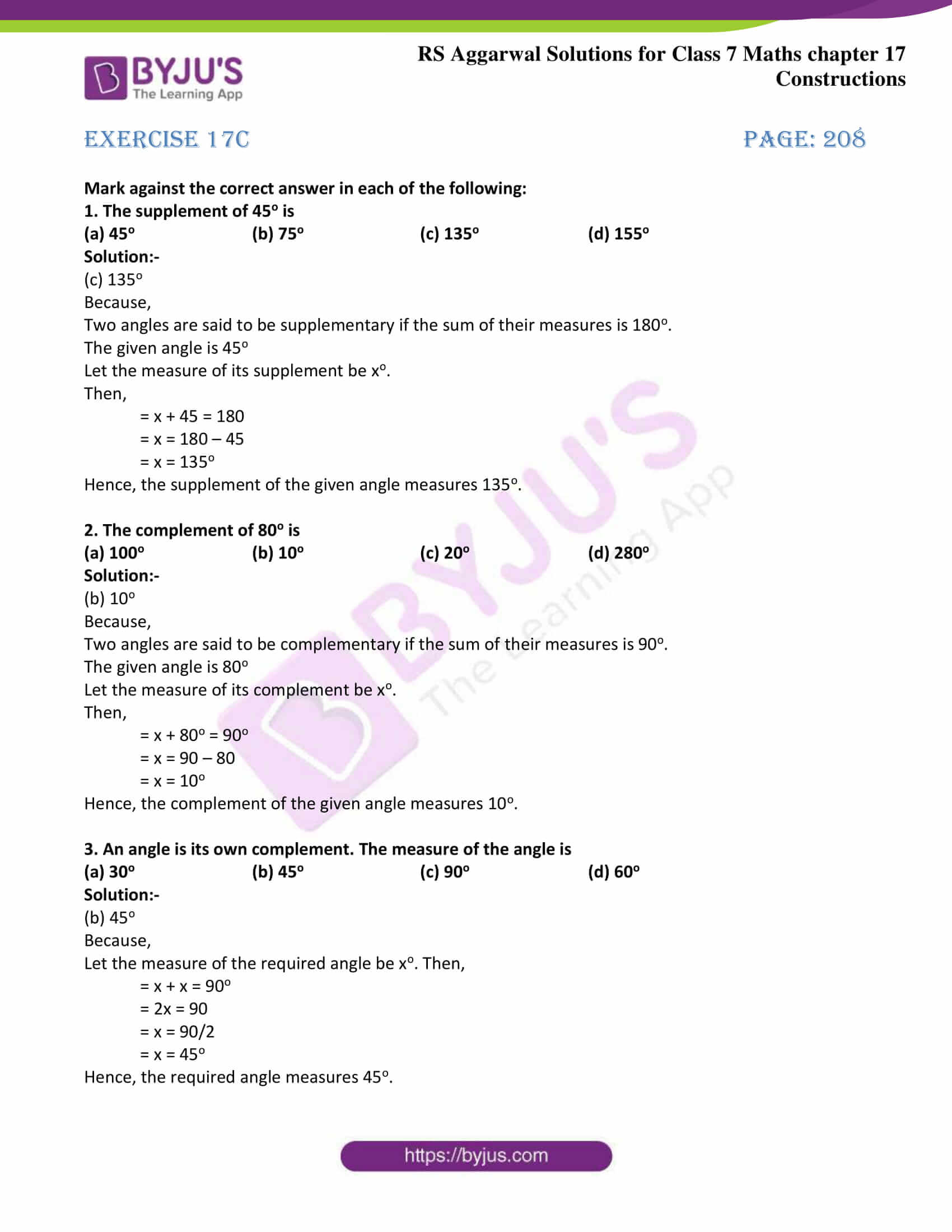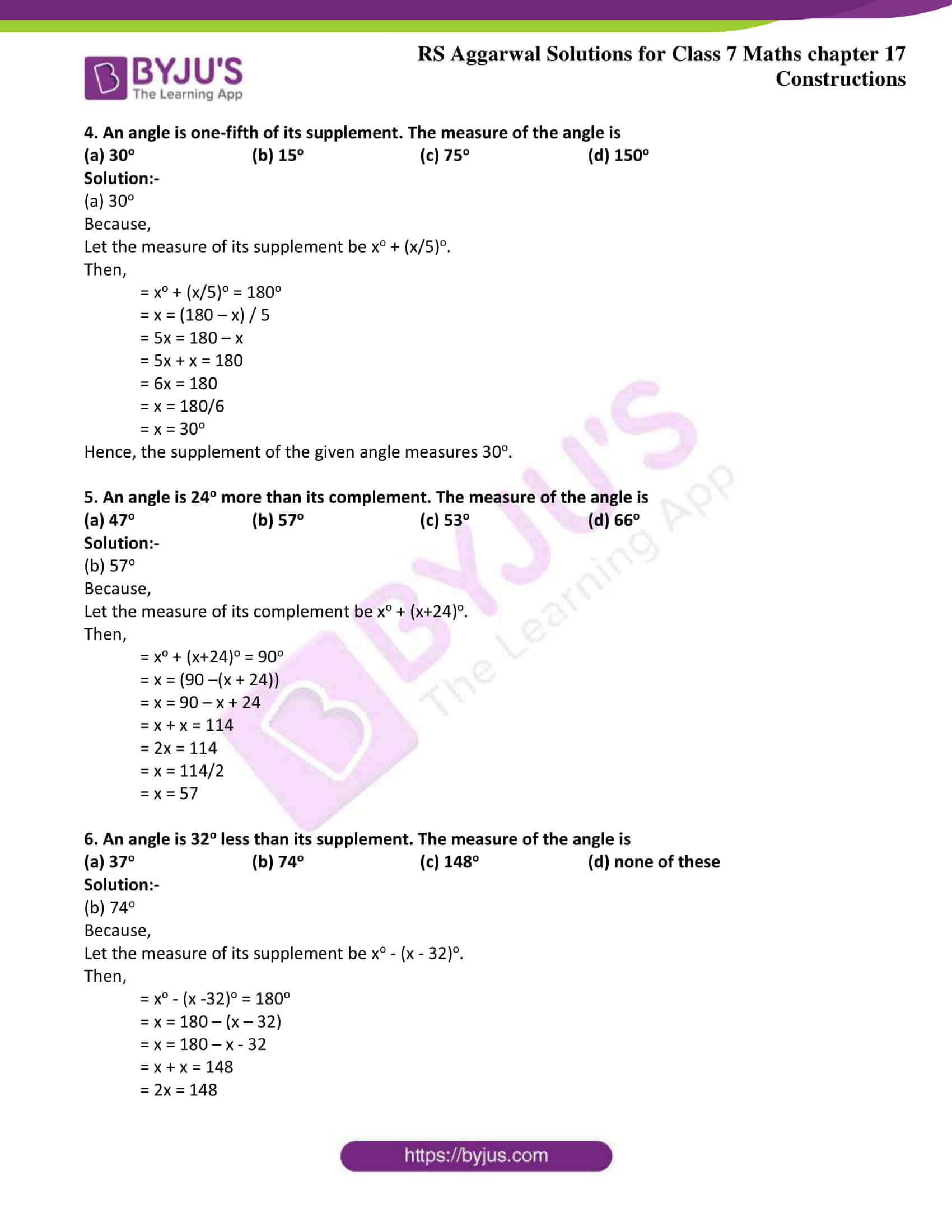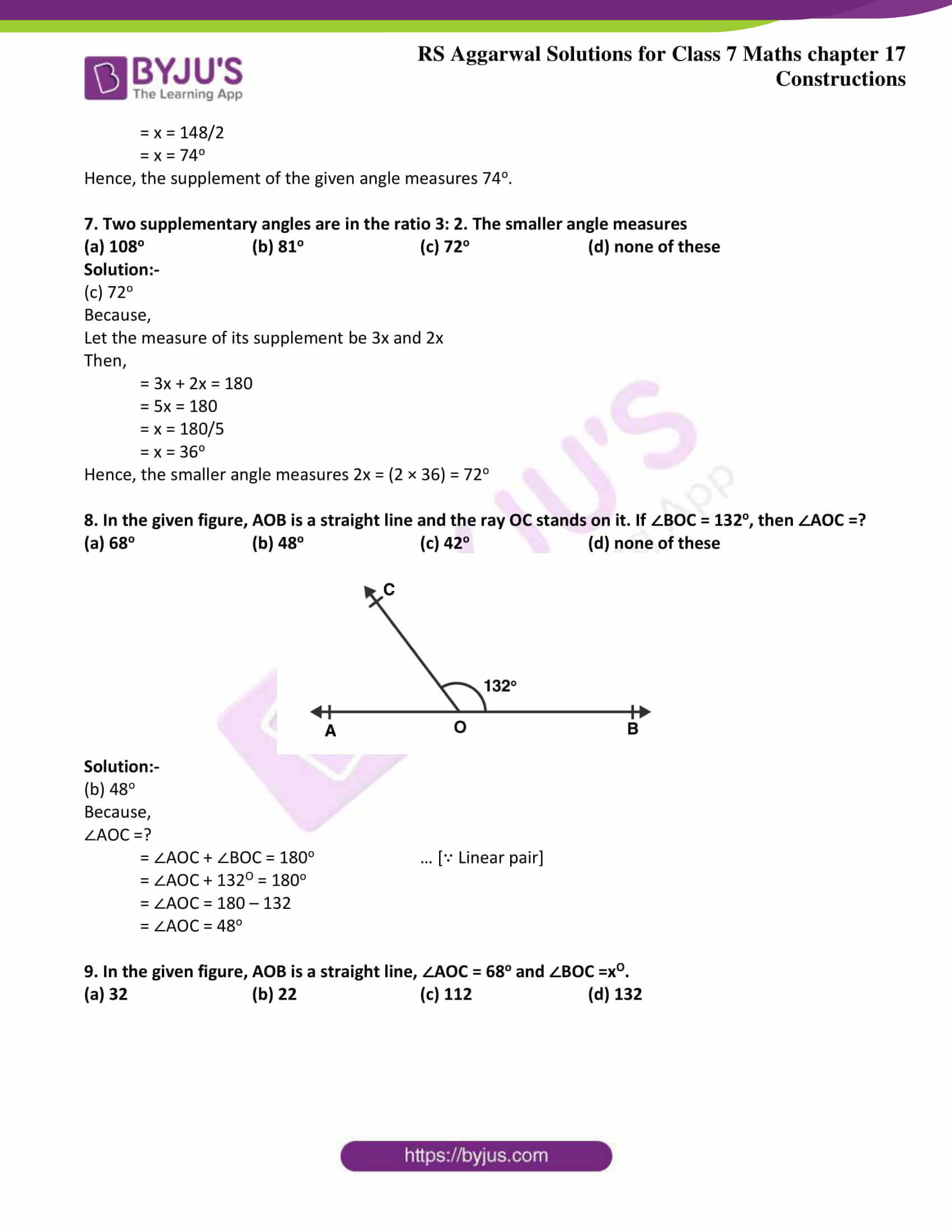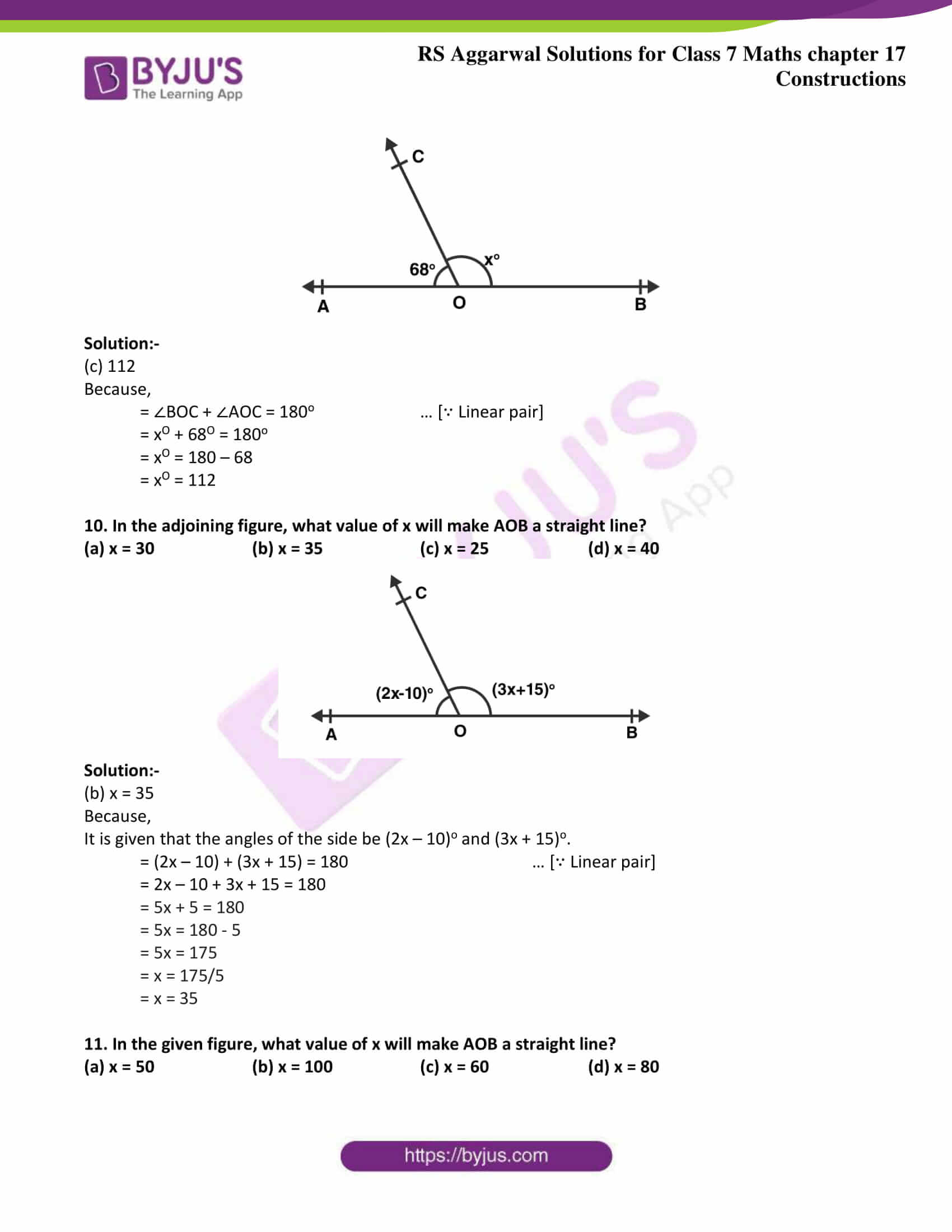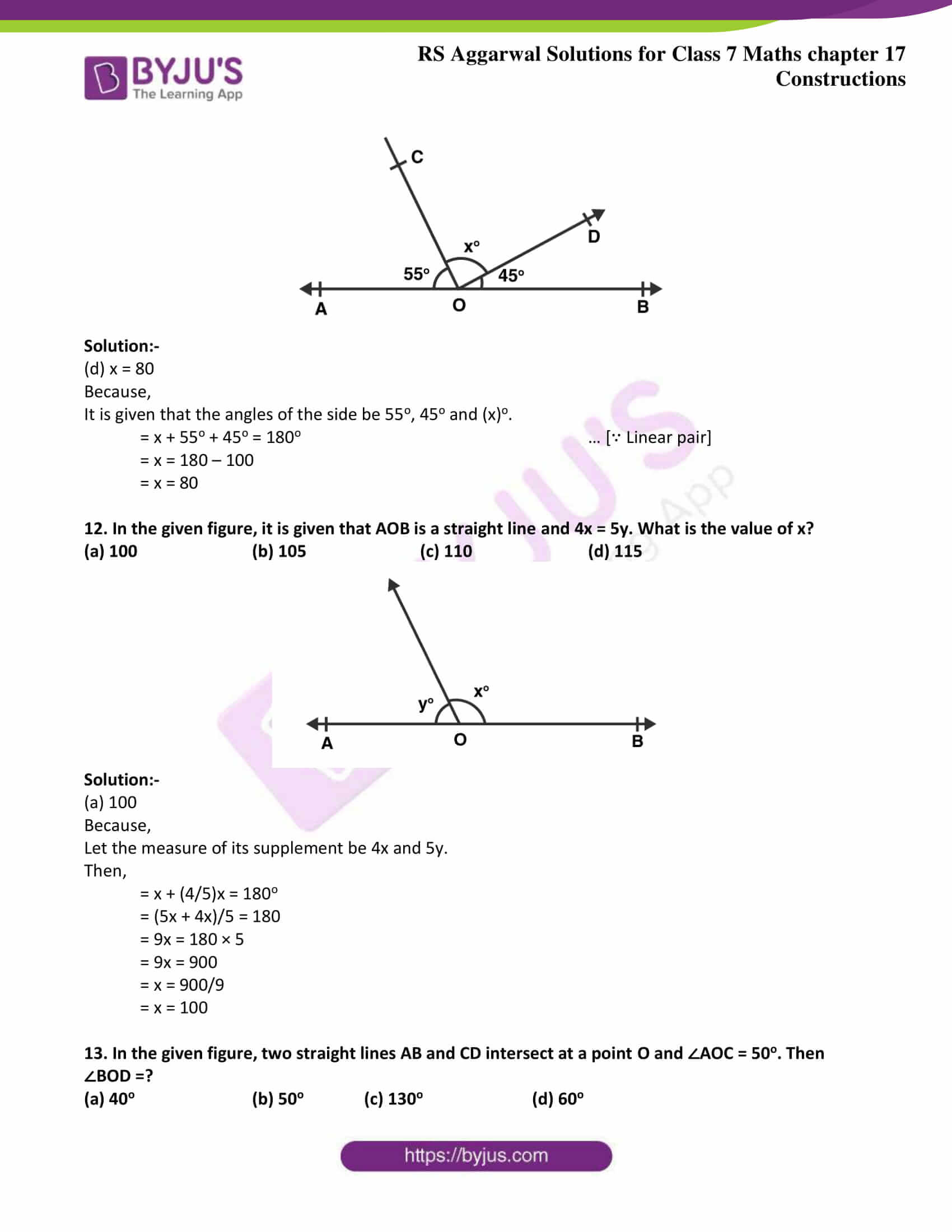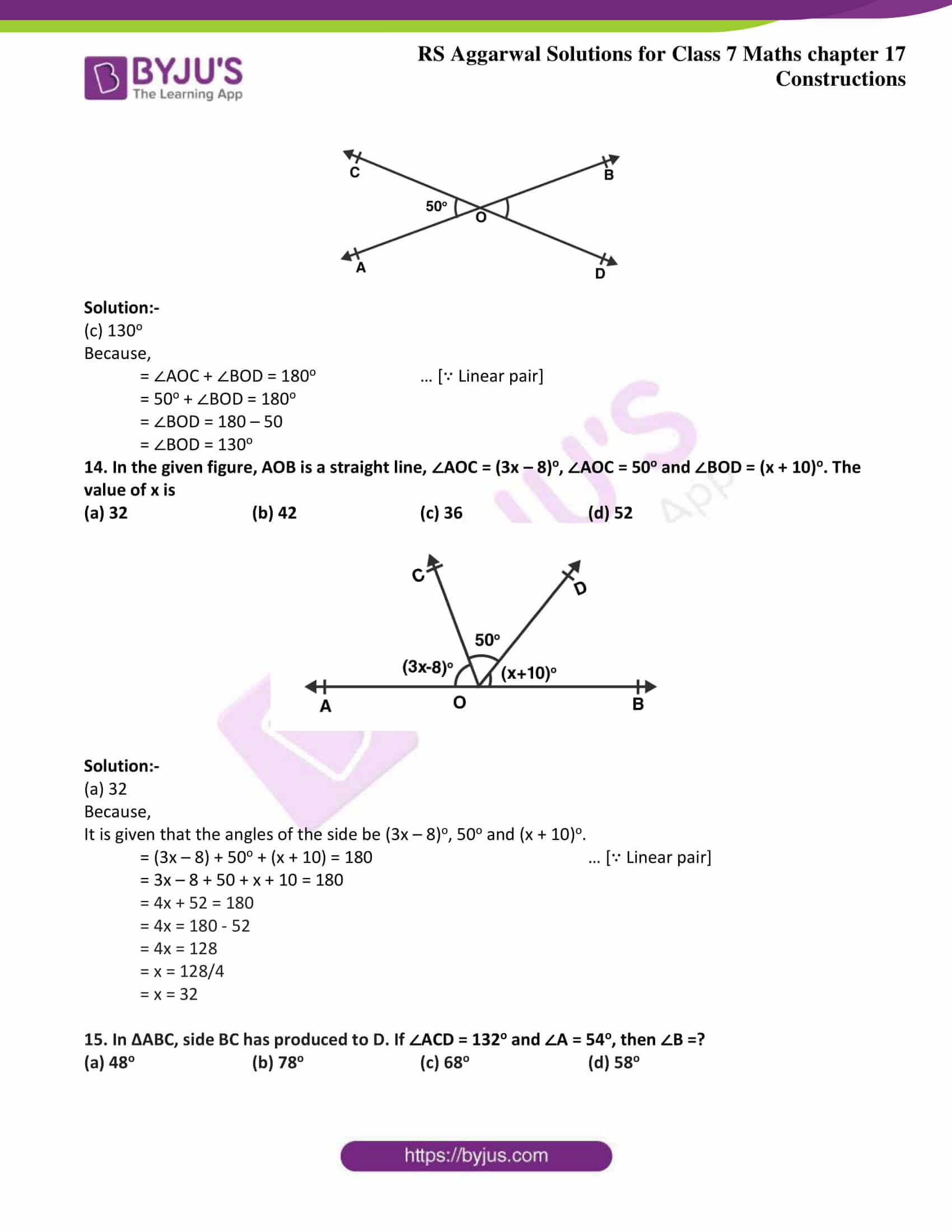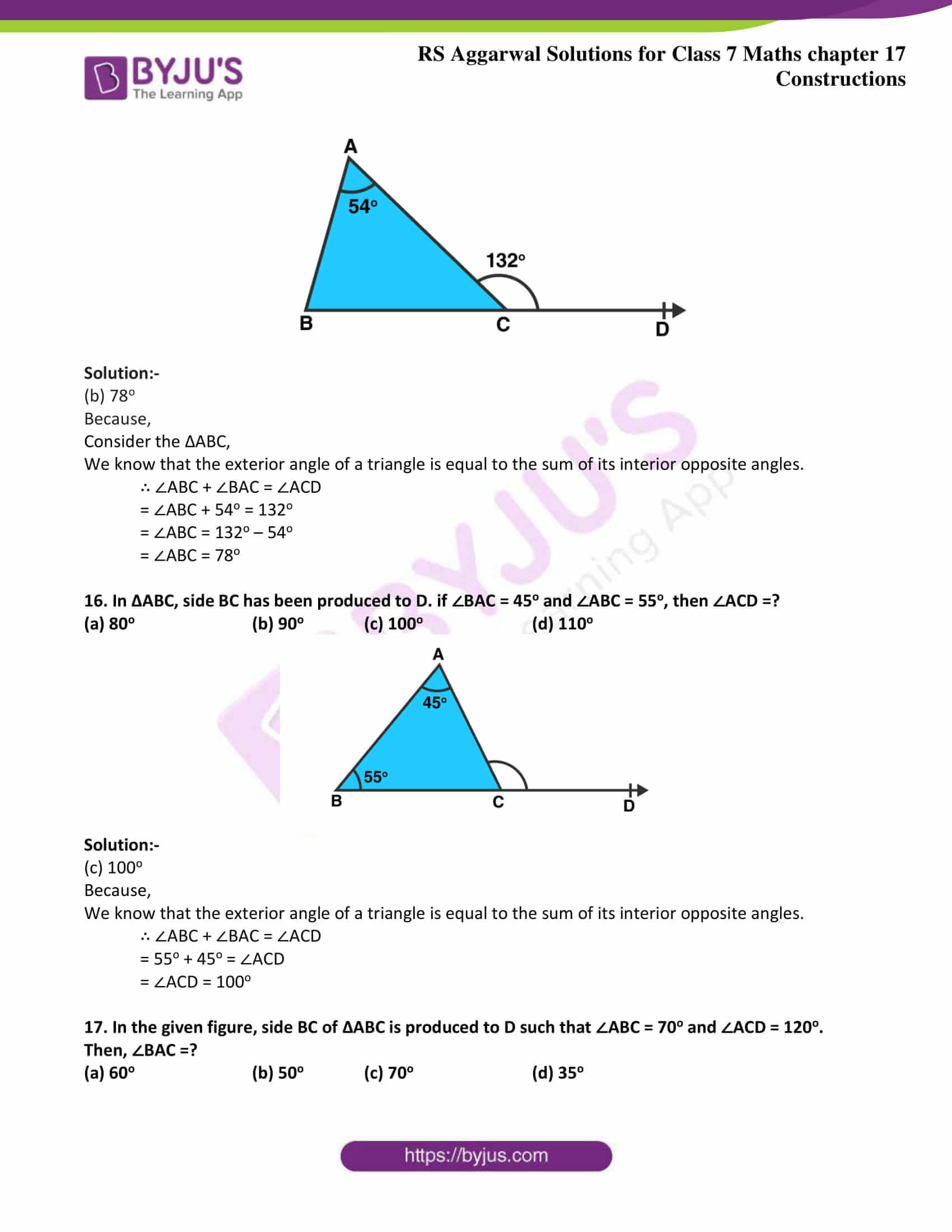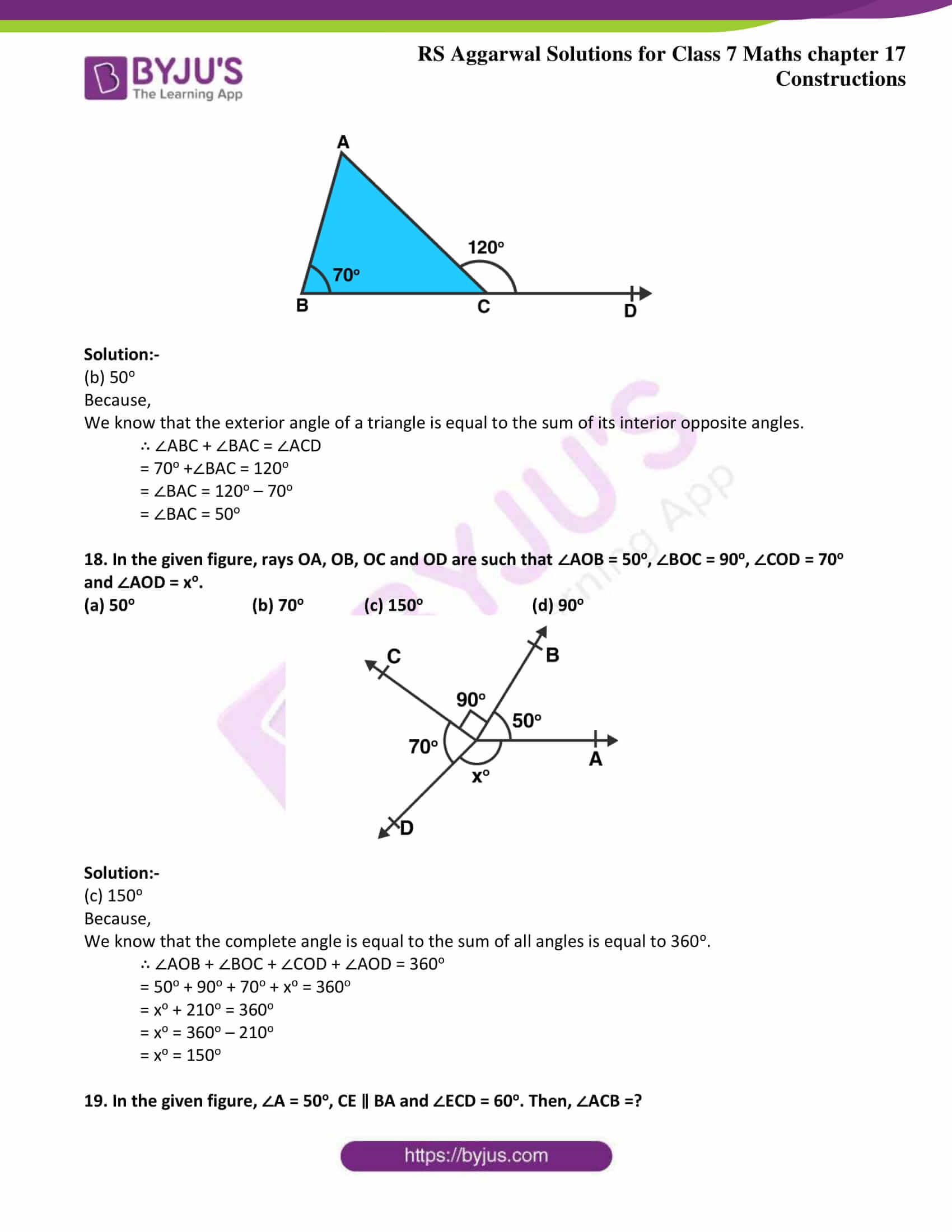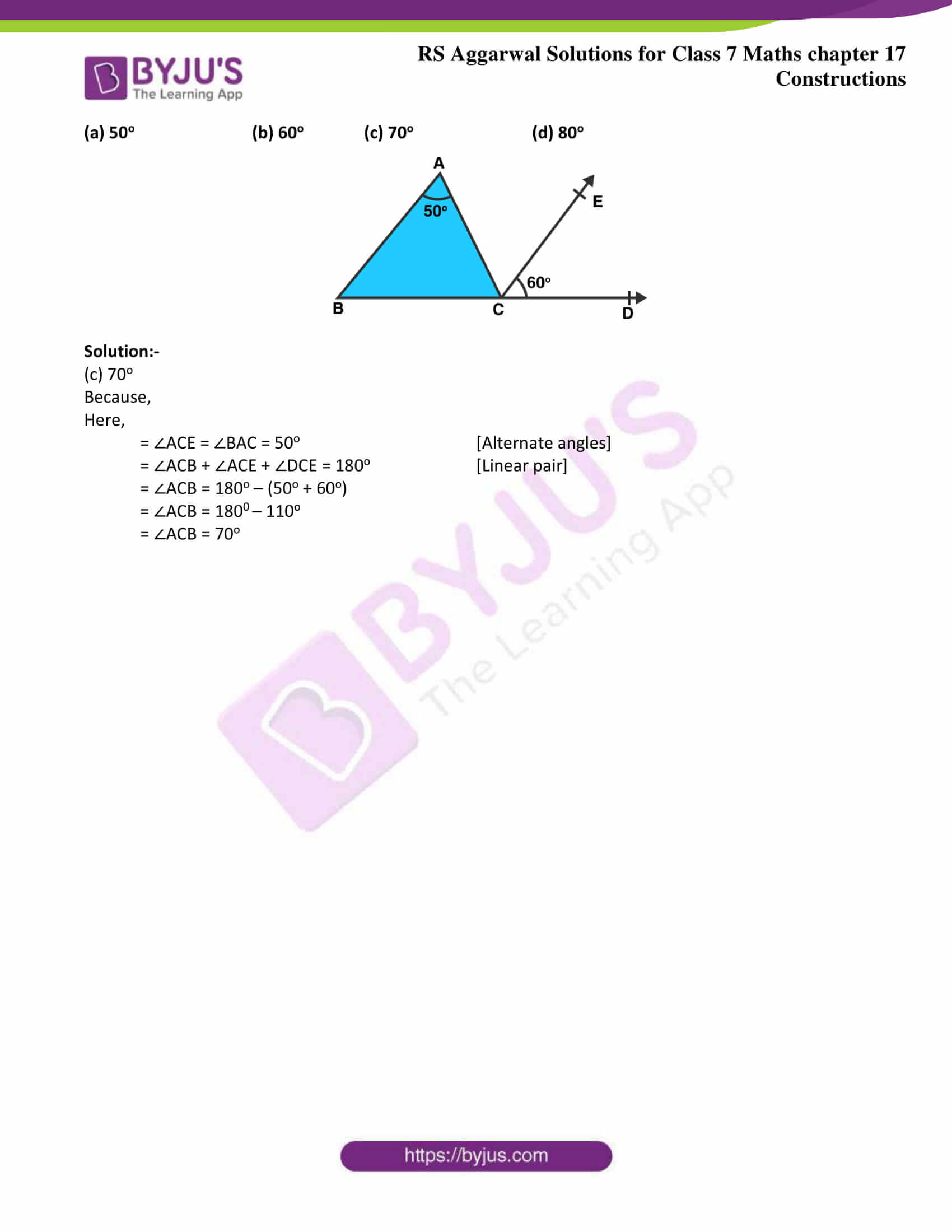Exercise 17A

Exercise 17B

Exercise 17C

## Exercise 17A Page: 204

1. Draw a line AB and take a point P outside it. Draw a line CD parallel to AB and passing through the point P.

Solution:-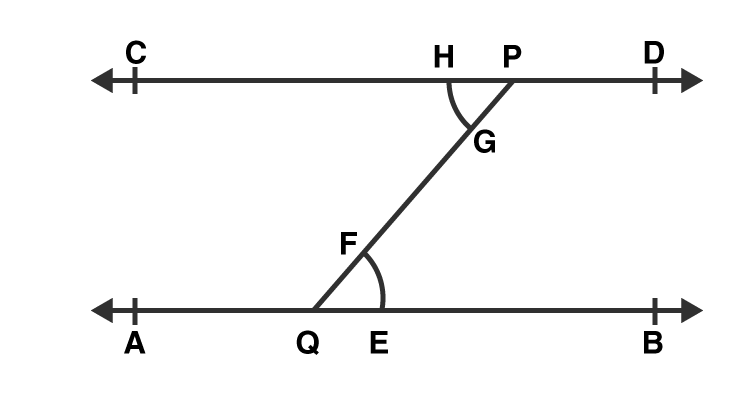Steps for construction,

1. Draw a line AB.

2. Take any point Q on AB and a point P outside AB and join PQ.

3. With Q as center and any radius draw an arc to cut AB at E and PQ at F.

4. With P as center and same radius draw an arc to cut QP at G.

5. With as center and radius equal to EF, draw an arc to cut the previous arc at H.

6. Join PH and produce it on both sides to get the required line CD parallel to AB.

2. Draw a line AB and draw another line CD parallel to AB at a distance of 3.5 cm from it.

Solution:-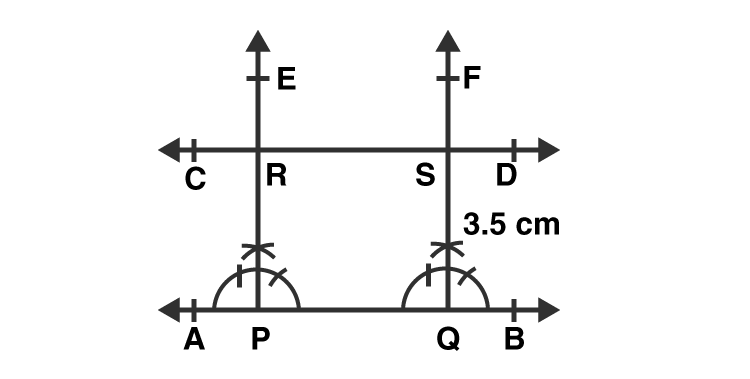Steps for construction,

1. Draw a line AB.

2. Take any two points P and Q on AB.

3. Construct ∠BPE = 90o and ∠BQF = 90o.

4. With P as center and radius equal to 3.5 cm, cut PE at R.

5. With Q as center and radius equal to 3.5 cm, cut QF at S.

6. Join CD and produce it on either side to get the required line CD parallel to AB and at a distance of 3 cm from it.

## Exercise 17B Page: 207

1. Construct a ΔABC in which BC = 3.6 cm, AB = 5 cm and AC = 5.4 cm. Draw the perpendicular bisector of the side BC.

Solution:-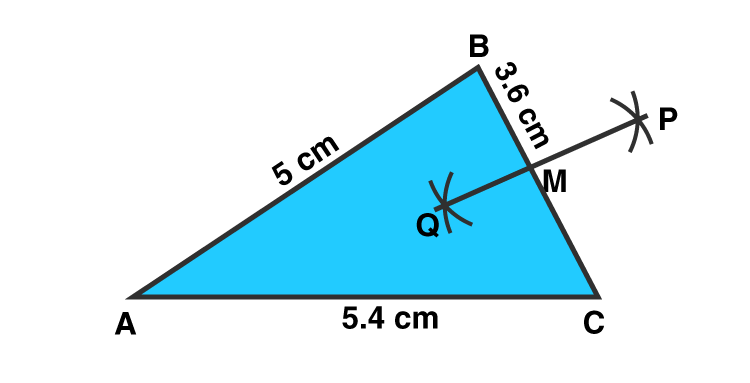Steps of construction:

1. Draw a line segment AC = 5.4 cm.

2. With A as a center and radius 5 cm, draw an arc.

3. With C as a center and radius 3.6 cm, draw another arc, cutting the previous arc at B.

4. Join AB and CB.

Then, ΔABC is the required triangle.

5. With B as center and radius measuring more than half of BC, draw arcs on both sides of BC.

6. With C as center and the same radius as before, draw arcs on both sides of BC, cutting the previous arcs at P and Q, as shown. Join PQ.

Then, PQ is the required perpendicular bisector of BC, meeting BC at M.

2. Construct a ΔPQR in which QR = 6 cm, PQ = 4.4 cm and PR = 5.3 cm. Draw the bisector of ∠P.

Solution:-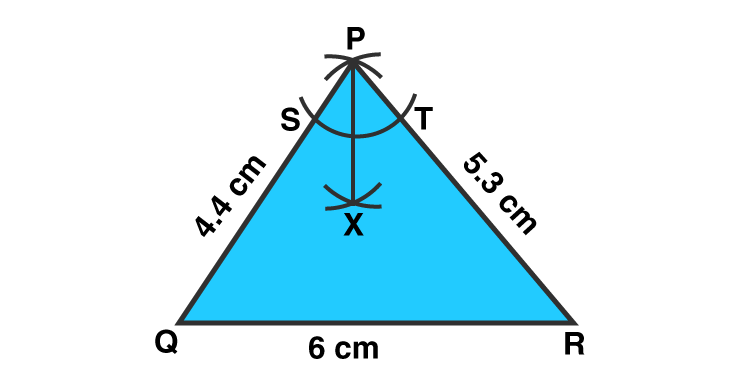Steps of construction:

1. Draw a line segment QR = 6 cm.

2. With Q as a center and radius 4.4 cm, draw an arc.

3. With R as a center and radius 5.3 cm, draw another arc, cutting the previous arc at P.

4. Join PQ and PR.

Then, ΔPQR is the required triangle.

5. With B as center and any radius cutting PQ and PR at S and T, respectively

6. With S as center and radius measuring more than half of ST, draw an arc.

7. With T as center and the same radius, draw another arc cutting the previous arc at x.

8. Join P and X

Then, PX is the required bisector of ∠P.

3. Construct an equilateral triangle each of whose sides measures 6.2 cm. Measures each one of its angles.

Solution:-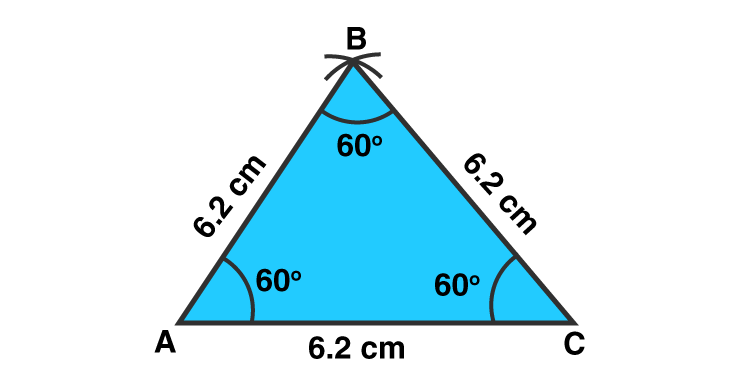Steps of construction:

1. Draw a line segment AC = 6.2 cm.

2. With A as a center and radius 6.2 cm, draw an arc.

3. With C as a center and radius 6.2 cm, draw another arc, cutting the previous arc at B.

4. Join AB and CB.

Then, ΔABC is the required equilateral triangle.

5. When we will measure all the angles of triangle by protractor, then all angles are equal to 60o.

4. Construct a ΔABC in which AB = AC = 4.8cm and BC = 5.3cm. Measure ∠B and ∠C. Draw AD ⊥ BC.

Solution:-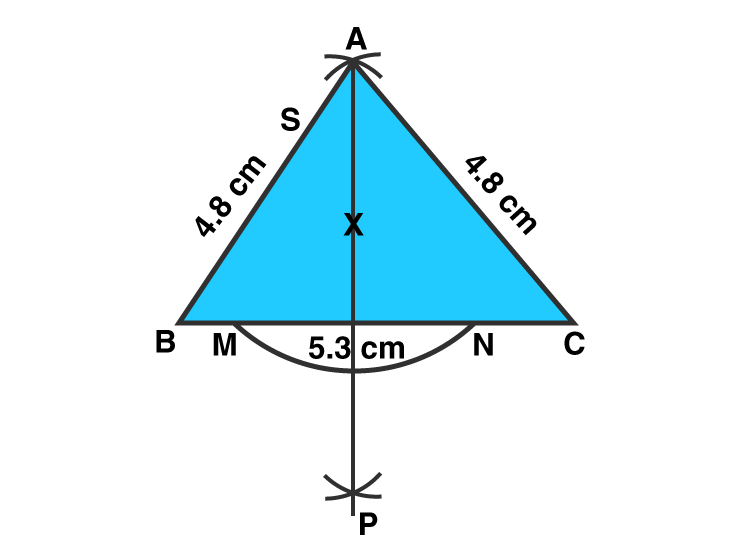Steps of construction:

1. Draw a line segment BC = 5.3 cm.

2. With B as a center and radius 4.8 cm, draw an arc.

3. With C as a center and radius 4.8 cm, draw another arc, cutting the previous arc at A.

4. Join AB and AC.

Then, ΔABC is the required triangle.

5. When we will measure ∠B and ∠C the angles of triangle by protractor, then the measure of angles are 56o and 56o respectively.

6. With A as the center and any radius, draw an arc cutting BC at M and N.

7. With M as the center and the radius more than half of MN, draw an arc.

8. With N as the center and the same radius, draw another arc cutting the previously drawn.

9. Join AP, cutting BC at D.

5. Construct a ΔABC in which AB = 3.8 cm, ∠A = 60o and AC = 5 cm.

Solution:-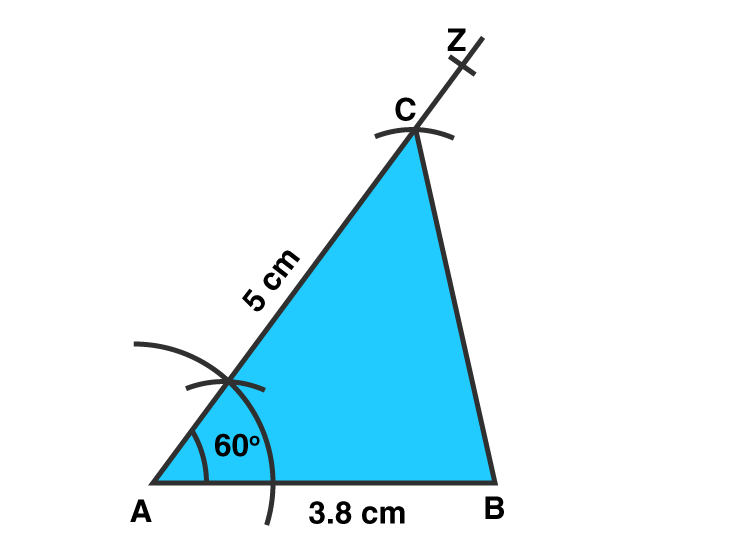Steps of construction:

1. Draw a line segment AB = 3.8 cm.

2. Construct ∠BAZ = 60o.

3. Along AZ, set off AC = 5cm.

4. Join BC.

Then, ΔABC is the required triangle.

6. Construct a ΔABC in which BC = 4.3 cm, ∠C = 45o and AC = 6 cm.

Solution:-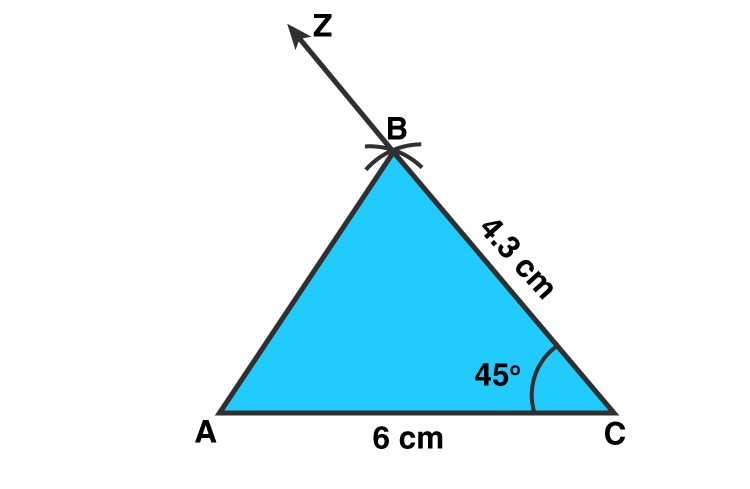Steps of construction:

1. Draw a line segment AC = 6 cm.

2. Construct ∠ACZ = 45o.

3. Along CZ, set off BC = 4.3cm.

4. Join BC.

Then, ΔABC is the required triangle.

## Exercise 17C Page: 208

Mark against the correct answer in each of the following:

1. The supplement of 45o is

(a) 45o (b) 75o (c) 135o (d) 155o

Solution:-

(c) 135o

Because,

Two angles are said to be supplementary if the sum of their measures is 180o.

The given angle is 45o

Let the measure of its supplement be xo.

Then,

= x + 45 = 180

= x = 180 – 45

= x = 135o

Hence, the supplement of the given angle measures 135o.

2. The complement of 80o is

(a) 100o (b) 10o (c) 20o (d) 280o

Solution:-

(b) 10o

Because,

Two angles are said to be complementary if the sum of their measures is 90o.

The given angle is 80o

Let the measure of its complement be xo.

Then,

= x + 80o = 90o

= x = 90 – 80

= x = 10o

Hence, the complement of the given angle measures 10o.

3. An angle is its own complement. The measure of the angle is

(a) 30o (b) 45o (c) 90o (d) 60o

Solution:-

(b) 45o

Because,

Let the measure of the required angle be xo. Then,

= x + x = 90o

= 2x = 90

= x = 90/2

= x = 45o

Hence, the required angle measures 45o.

4. An angle is one-fifth of its supplement. The measure of the angle is

(a) 30o (b) 15o (c) 75o (d) 150o

Solution:-

(a) 30o

Because,

Let the measure of its supplement be xo + (x/5)o.

Then,

= xo + (x/5)o = 180o

= x = (180 – x) / 5

= 5x = 180 – x

= 5x + x = 180

= 6x = 180

= x = 180/6

= x = 30o

Hence, the supplement of the given angle measures 30o.

5. An angle is 24o more than its complement. The measure of the angle is

(a) 47o (b) 57o (c) 53o (d) 66o

Solution:-

(b) 57o

Because,

Let the measure of its complement be xo + (x+24)o.

Then,

= xo + (x+24)o = 90o

= x = (90 –(x + 24))

= x = 90 – x + 24

= x + x = 114

= 2x = 114

= x = 114/2

= x = 57

6. An angle is 32o less than its supplement. The measure of the angle is

(a) 37o (b) 74o (c) 148o (d) none of these

Solution:-

(b) 74o

Because,

Let the measure of its supplement be xo – (x – 32)o.

Then,

= xo – (x -32)o = 180o

= x = 180 – (x – 32)

= x = 180 – x – 32

= x + x = 148

= 2x = 148

= x = 148/2

= x = 74o

Hence, the supplement of the given angle measures 74o.

7. Two supplementary angles are in the ratio 3: 2. The smaller angle measures

(a) 108o (b) 81o (c) 72o (d) none of these

Solution:-

(c) 72o

Because,

Let the measure of its supplement be 3x and 2x

Then,

= 3x + 2x = 180

= 5x = 180

= x = 180/5

= x = 36o

Hence, the smaller angle measures 2x = (2 × 36) = 72o

8. In the given figure, AOB is a straight line and the ray OC stands on it. If ∠BOC = 132o, then ∠AOC =?

(a) 68o (b) 48o (c) 42o (d) none of these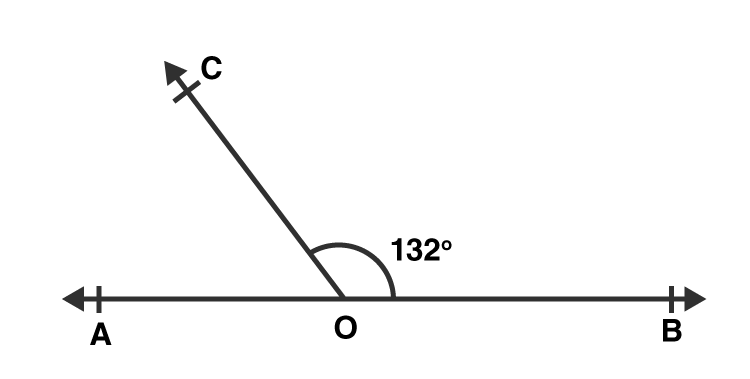Solution:-

(b) 48o

Because,

∠AOC =?

= ∠AOC + ∠BOC = 180o … [∵ Linear pair]

= ∠AOC + 132O = 180o

= ∠AOC = 180 – 132

= ∠AOC = 48o

9. In the given figure, AOB is a straight line, ∠AOC = 68o and ∠BOC =xO.

(a) 32 (b) 22 (c) 112 (d) 132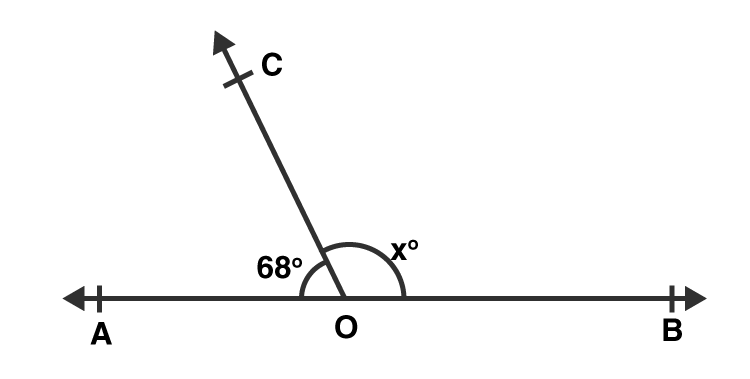Solution:-

(c) 112

Because,

= ∠BOC + ∠AOC = 180o … [∵ Linear pair]

= xO + 68O = 180o

= xO = 180 – 68

= xO = 112

10. In the adjoining figure, what value of x will make AOB a straight line?

(a) x = 30 (b) x = 35 (c) x = 25 (d) x = 40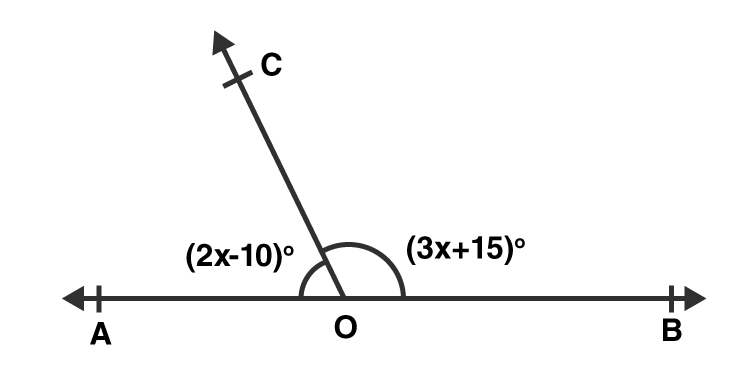Solution:-

(b) x = 35

Because,

It is given that the angles of the side be (2x – 10)o and (3x + 15)o.

= (2x – 10) + (3x + 15) = 180 … [∵ Linear pair]

= 2x – 10 + 3x + 15 = 180

= 5x + 5 = 180

= 5x = 180 – 5

= 5x = 175

= x = 175/5

= x = 35

11. In the given figure, what value of x will make AOB a straight line?

(a) x = 50 (b) x = 100 (c) x = 60 (d) x = 80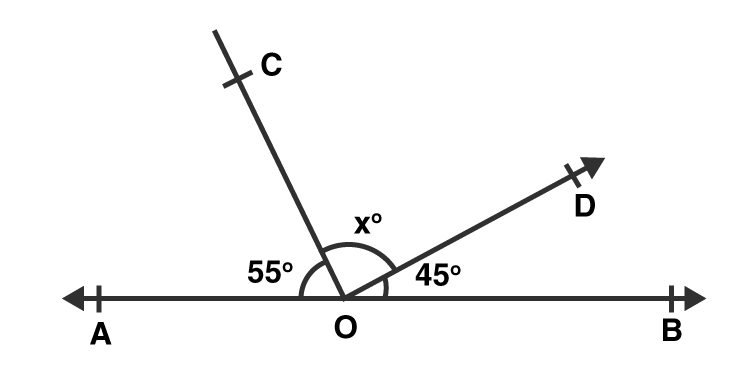Solution:-

(d) x = 80

Because,

It is given that the angles of the side be 55o, 45o and (x)o.

= x + 55o + 45o = 180o … [∵ Linear pair]

= x = 180 – 100

= x = 80

12. In the given figure, it is given that AOB is a straight line and 4x = 5y. What is the value of x?

(a) 100 (b) 105 (c) 110 (d) 115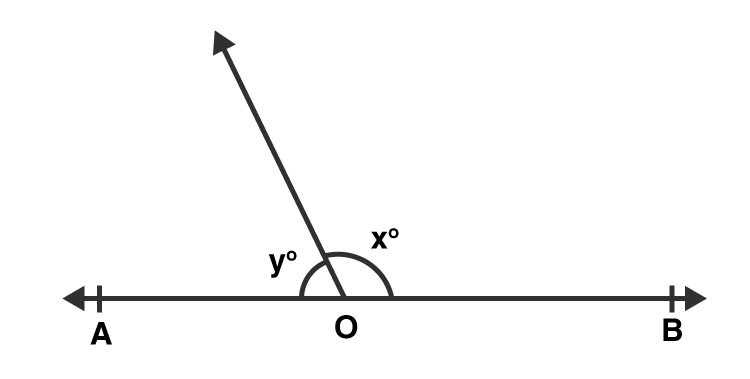Solution:-

(a) 100

Because,

Let the measure of its supplement be 4x and 5y.

Then,

= x + (4/5)x = 180o

= (5x + 4x)/5 = 180

= 9x = 180 × 5

= 9x = 900

= x = 900/9

= x = 100

13. In the given figure, two straight lines AB and CD intersect at a point O and ∠AOC = 50o. Then ∠BOD =?

(a) 40o (b) 50o (c) 130o (d) 60o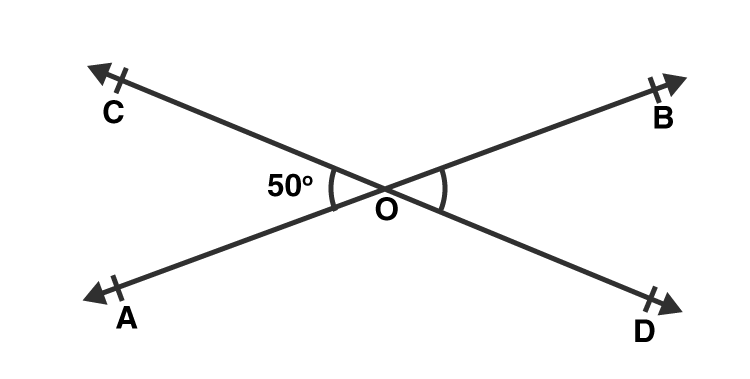Solution:-

(c) 130o

Because,

= ∠AOC + ∠BOD = 180o … [∵ Linear pair]

= 50o + ∠BOD = 180o

= ∠BOD = 180 – 50

= ∠BOD = 130o

14. In the given figure, AOB is a straight line, ∠AOC = (3x – 8)o, ∠AOC = 50o and ∠BOD = (x + 10)o. The value of x is

(a) 32 (b) 42 (c) 36 (d) 52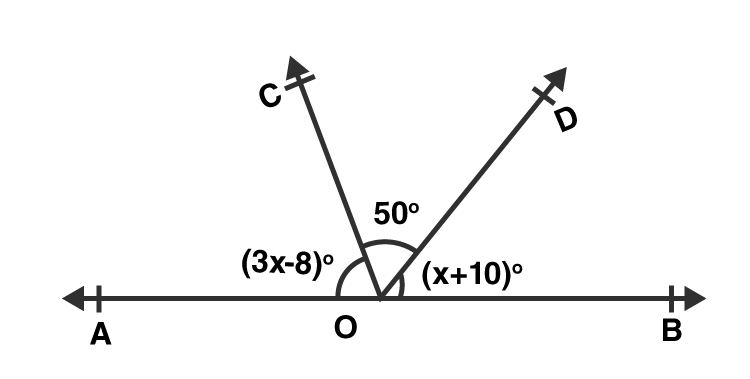Solution:-

(a) 32

Because,

It is given that the angles of the side be (3x – 8)o, 50o and (x + 10)o.

= (3x – 8) + 50o + (x + 10) = 180 … [∵ Linear pair]

= 3x – 8 + 50 + x + 10 = 180

= 4x + 52 = 180

= 4x = 180 – 52

= 4x = 128

= x = 128/4

= x = 32

15. In ΔABC, side BC has produced to D. If ∠ACD = 132o and ∠A = 54o, then ∠B =?

(a) 48o (b) 78o (c) 68o (d) 58o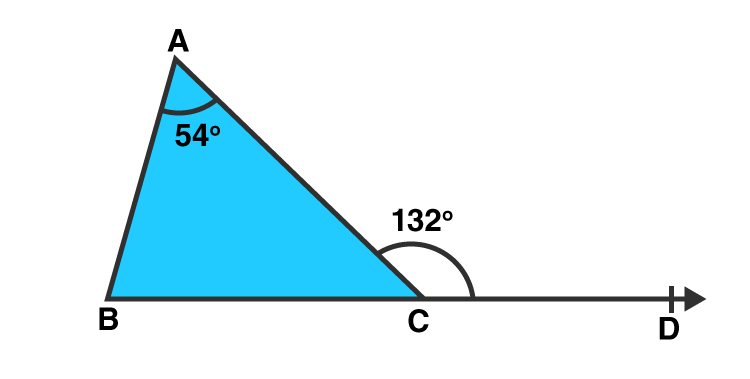Solution:-

(b) 78o

Because,

Consider the ΔABC,

We know that the exterior angle of a triangle is equal to the sum of its interior opposite angles.

∴ ∠ABC + ∠BAC = ∠ACD

= ∠ABC + 54o = 132o

= ∠ABC = 132o – 54o

= ∠ABC = 78o

16. In ΔABC, side BC has been produced to D. if ∠BAC = 45o and ∠ABC = 55o, then ∠ACD =?

(a) 80o (b) 90o (c) 100o (d) 110o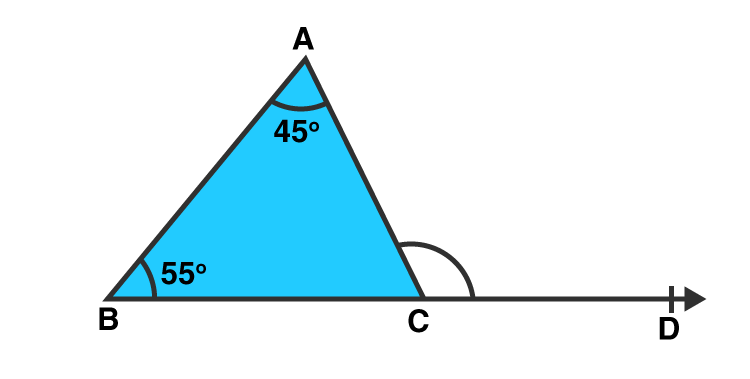Solution:-

(c) 100o

Because,

We know that the exterior angle of a triangle is equal to the sum of its interior opposite angles.

∴ ∠ABC + ∠BAC = ∠ACD

= 55o + 45o = ∠ACD

= ∠ACD = 100o

17. In the given figure, side BC of ΔABC is produced to D such that ∠ABC = 70o and ∠ACD = 120o. Then, ∠BAC =?

(a) 60o (b) 50o (c) 70o (d) 35o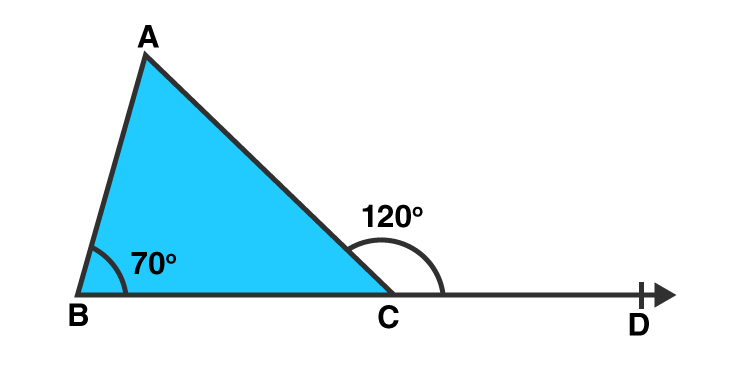Solution:-

(b) 50o

Because,

We know that the exterior angle of a triangle is equal to the sum of its interior opposite angles.

∴ ∠ABC + ∠BAC = ∠ACD

= 70o +∠BAC = 120o

= ∠BAC = 120o – 70o

= ∠BAC = 50o

18. In the given figure, rays OA, OB, OC and OD are such that ∠AOB = 50o, ∠BOC = 90o, ∠COD = 70o and ∠AOD = xo.

(a) 50o (b) 70o (c) 150o (d) 90o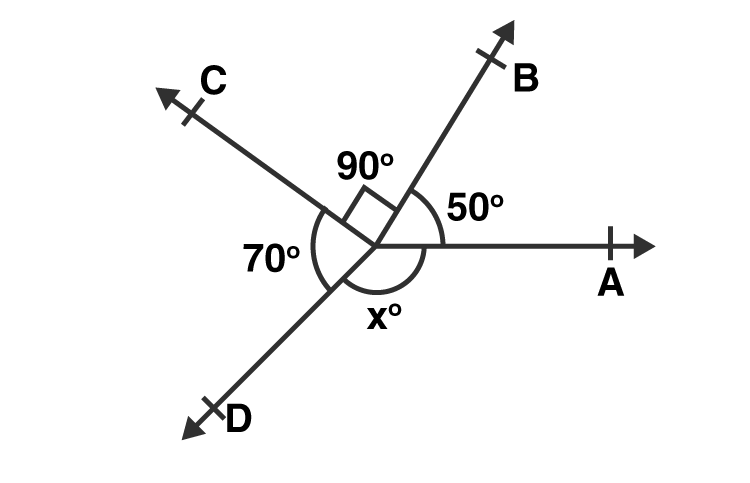Solution:-

(c) 150o

Because,

We know that the complete angle is equal to the sum of all angles is equal to 360o.

∴ ∠AOB + ∠BOC + ∠COD + ∠AOD = 360o

= 50o + 90o + 70o + xo = 360o

= xo + 210o = 360o

= xo = 360o – 210o

= xo = 150o

19. In the given figure, ∠A = 50o, CE ∥ BA and ∠ECD = 60o. Then, ∠ACB =?

(a) 50o (b) 60o (c) 70o (d) 80o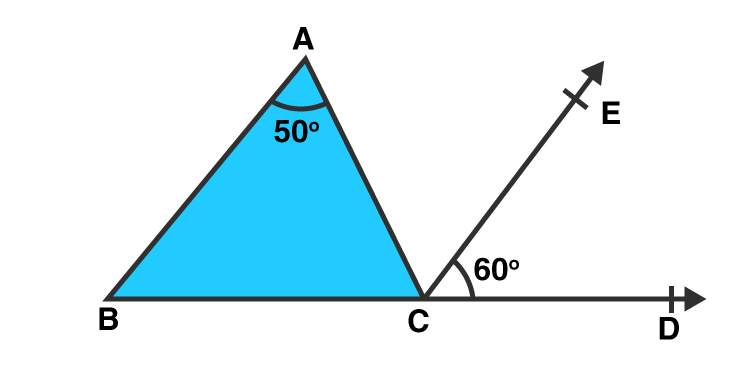Solution:-

(c) 70o

Because,

Here,

= ∠ACE = ∠BAC = 50o [Alternate angles]

= ∠ACB + ∠ACE + ∠DCE = 180o [Linear pair]

= ∠ACB = 180o – (50o + 60o)

= ∠ACB = 1800 – 110o

= ∠ACB = 70o

## RS Aggarwal Solutions for Class 7 Maths Chapter 17 – Constructions

Chapter 17 – Constructions contains 3 exercises and the RS Aggarwal Solutions available on this page provide solutions to the questions present in the exercises. Now, let us have a look at some of the concepts discussed in this chapter.

• Construction of Parallel Lines
• Construction of Triangles
• SSS Triangle Construction
• SAS Triangle Construction
• ASA Triangle Construction
• RHS Triangle Construction

### Chapter Brief of RS Aggarwal Solutions for Class 7 Maths Chapter 17 – Constructions

RS Aggarwal Solutions for Class 7 Maths Chapter 17 – Constructions refers to a precise way of drawing using two tools, an unmarked straight line and a compass. In this chapter, students are going to learn about the construction of parallel lines and the construction of different types of triangles.